Courses

# Paper Folding Quiz I, Non Verbal Reasoning

## 20 Questions MCQ Test General Intelligence and Reasoning for SSC CGL | Paper Folding Quiz I, Non Verbal Reasoning

Description
This mock test of Paper Folding Quiz I, Non Verbal Reasoning for LR helps you for every LR entrance exam. This contains 20 Multiple Choice Questions for LR Paper Folding Quiz I, Non Verbal Reasoning (mcq) to study with solutions a complete question bank. The solved questions answers in this Paper Folding Quiz I, Non Verbal Reasoning quiz give you a good mix of easy questions and tough questions. LR students definitely take this Paper Folding Quiz I, Non Verbal Reasoning exercise for a better result in the exam. You can find other Paper Folding Quiz I, Non Verbal Reasoning extra questions, long questions & short questions for LR on EduRev as well by searching above.
QUESTION: 1

Solution:
QUESTION: 2

Solution:
QUESTION: 3

### Directions to Solve In each of the following problems, a square transparent sheet (X) with a pattern is given. Figure out from amongst the four alternatives as to how the patter would appear when the transparent sheet is folded at the dotted line. Question - Find out from amongst the four alternatives as to how the pattern would appear when the transparent sheet is folded at the dotted line.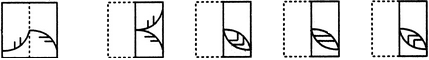(X)                     (1)                 (2)                 (3)                 (4)

Solution:
QUESTION: 4

In each of the following problems, a square transparent sheet (X) with a pattern is given. Figure out from amongst the four alternatives as to how the patter would appear when the transparent sheet is folded at the dotted line.

Question -

Find out from amongst the four alternatives as to how the pattern would appear when the transparent sheet is folded at the dotted line.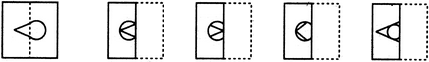(X)                     (1)                 (2)                 (3)                 (4)
Solution:
QUESTION: 5

In each of the following problems, a square transparent sheet (X) with a pattern is given. Figure out from amongst the four alternatives as to how the patter would appear when the transparent sheet is folded at the dotted line.

Question -

Find out from amongst the four alternatives as to how the pattern would appear when the transparent sheet is folded at the dotted line.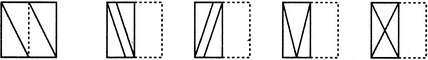(X)                     (1)                 (2)                 (3)                 (4)
Solution:
QUESTION: 6

In each of the following problems, a square transparent sheet (X) with a pattern is given. Figure out from amongst the four alternatives as to how the patter would appear when the transparent sheet is folded at the dotted line.

Question -

Find out from amongst the four alternatives as to how the pattern would appear when the transparent sheet is folded at the dotted line.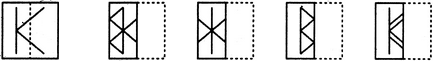(X)                     (1)                 (2)                 (3)                 (4)
Solution:
QUESTION: 7

In each of the following problems, a square transparent sheet (X) with a pattern is given. Figure out from amongst the four alternatives as to how the patter would appear when the transparent sheet is folded at the dotted line.

Question -

Find out from amongst the four alternatives as to how the pattern would appear when the transparent sheet is folded at the dotted line.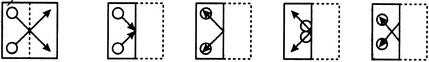(X)                     (1)                 (2)                 (3)                 (4)
Solution:
QUESTION: 8

In each of the following problems, a square transparent sheet (X) with a pattern is given. Figure out from amongst the four alternatives as to how the patter would appear when the transparent sheet is folded at the dotted line.

Question -

Find out from amongst the four alternatives as to how the pattern would appear when the transparent sheet is folded at the dotted line.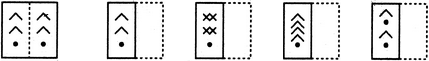(X)                     (1)                 (2)                 (3)                 (4)
Solution:
QUESTION: 9

In each of the following problems, a square transparent sheet (X) with a pattern is given. Figure out from amongst the four alternatives as to how the patter would appear when the transparent sheet is folded at the dotted line.

Question -

Find out from amongst the four alternatives as to how the pattern would appear when the transparent sheet is folded at the dotted line.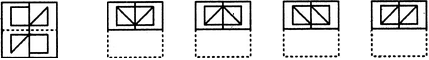(X)                     (1)                 (2)                 (3)                 (4)
Solution:
QUESTION: 10

In each of the following problems, a square transparent sheet (X) with a pattern is given. Figure out from amongst the four alternatives as to how the patter would appear when the transparent sheet is folded at the dotted line.

Question -

Find out from amongst the four alternatives as to how the pattern would appear when the transparent sheet is folded at the dotted line.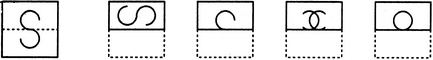(X)                     (1)                 (2)                 (3)                 (4)
Solution:
QUESTION: 11

In each of the following problems, a square transparent sheet (X) with a pattern is given. Figure out from amongst the four alternatives as to how the patter would appear when the transparent sheet is folded at the dotted line.

Question -

Find out from amongst the four alternatives as to how the pattern would appear when the transparent sheet is folded at the dotted line.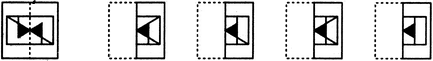(X)                     (1)                 (2)                 (3)                 (4)
Solution:
QUESTION: 12

In each of the following problems, a square transparent sheet (X) with a pattern is given. Figure out from amongst the four alternatives as to how the patter would appear when the transparent sheet is folded at the dotted line.

Question -

Find out from amongst the four alternatives as to how the pattern would appear when the transparent sheet is folded at the dotted line.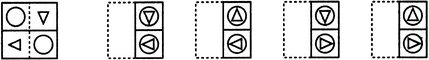(X)                     (1)                 (2)                 (3)                 (4)
Solution:
QUESTION: 13

In each of the following problems, a square transparent sheet (X) with a pattern is given. Figure out from amongst the four alternatives as to how the patter would appear when the transparent sheet is folded at the dotted line.

Question -

Find out from amongst the four alternatives as to how the pattern would appear when the transparent sheet is folded at the dotted line.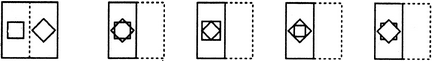(X)                     (1)                 (2)                 (3)                 (4)
Solution:
QUESTION: 14

In each of the following problems, a square transparent sheet (X) with a pattern is given. Figure out from amongst the four alternatives as to how the patter would appear when the transparent sheet is folded at the dotted line.

Question -

Find out from amongst the four alternatives as to how the pattern would appear when the transparent sheet is folded at the dotted line.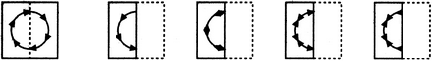(X)                     (1)                 (2)                 (3)                 (4)
Solution:
QUESTION: 15

In each of the following problems, a square transparent sheet (X) with a pattern is given. Figure out from amongst the four alternatives as to how the patter would appear when the transparent sheet is folded at the dotted line.

Question -

Find out from amongst the four alternatives as to how the pattern would appear when the transparent sheet is folded at the dotted line.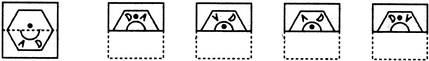(X)                     (1)                 (2)                 (3)                 (4)
Solution:
QUESTION: 16

In each of the following problems, a square transparent sheet (X) with a pattern is given. Figure out from amongst the four alternatives as to how the patter would appear when the transparent sheet is folded at the dotted line.

Question -

Find out from amongst the four alternatives as to how the pattern would appear when the transparent sheet is folded at the dotted line.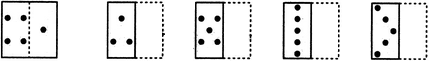(X)                     (1)                 (2)                 (3)                 (4)
Solution:
QUESTION: 17

In each of the following problems, a square transparent sheet (X) with a pattern is given. Figure out from amongst the four alternatives as to how the patter would appear when the transparent sheet is folded at the dotted line.

Question -

Find out from amongst the four alternatives as to how the pattern would appear when the transparent sheet is folded at the dotted line.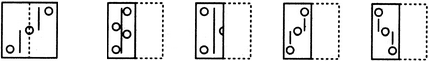(X)                     (1)                 (2)                 (3)                 (4)
Solution:
QUESTION: 18

In each of the following problems, a square transparent sheet (X) with a pattern is given. Figure out from amongst the four alternatives as to how the patter would appear when the transparent sheet is folded at the dotted line.

Question -

Find out from amongst the four alternatives as to how the pattern would appear when the transparent sheet is folded at the dotted line.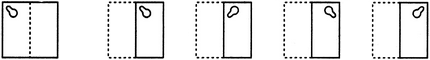(X)                     (1)                 (2)                 (3)                 (4)
Solution:
QUESTION: 19

In each of the following problems, a square transparent sheet (X) with a pattern is given. Figure out from amongst the four alternatives as to how the patter would appear when the transparent sheet is folded at the dotted line.

Question -

Find out from amongst the four alternatives as to how the pattern would appear when the transparent sheet is folded at the dotted line.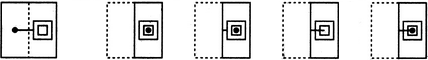(X)                     (1)                 (2)                 (3)                 (4)
Solution:
QUESTION: 20

Directions to Solve

In each of the following problems, a square transparent sheet (X) with a pattern is given. Figure out from amongst the four alternatives as to how the patter would appear when the transparent sheet is folded at the dotted line.

Question -

Find out from amongst the four alternatives as to how the pattern would appear when the transparent sheet is folded at the dotted line.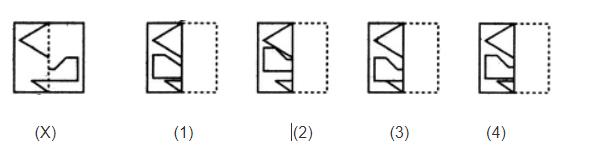Solution: